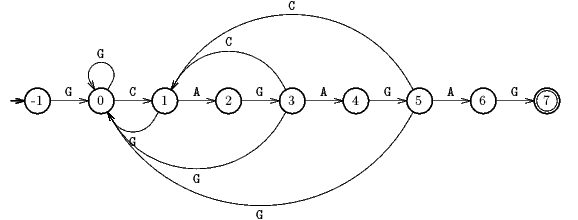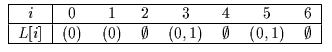Next: Colussi algorithm Up: ESMAJ Prev: Knuth-Morris-Pratt algorithm

# Simon algorithm

Main features
• economical implementation of  A(x) the minimal Deterministic Finite Automaton recognizing*x;
• preprocessing phase in O(m) time and space complexity;
• searching phase in O(m+n) time complexity (independent from the alphabet size);
• at most 2n-1 text character comparisons during the searching phase;
• delay bounded by min{1 + log2m,}.
Description

The main drawback of the search with the minimal  A(x) (see Deterministic Finite Automaton) is the size of the automaton: O(m).

Simon noticed that there are only a few significant edges in  A(x) ;

they are:the forward edges going from the prefix of x of length k to the prefix of length k+1 for 0k < m. There are exactly m such edges;the backward edges going from the prefix of x of length k to a smaller non-zero length prefix. The number of such edges is bounded by m.

The other edges are leading to the initial state and can then be deduced. Thus the number of significant edges is bounded by 2m. Then for each state of the automaton it is only necessary to store the list of its significant outgoing edges.

Each state is represented by the length of its associated prefix minus 1 in order that each edge leading to state i, with -1im-1 is labelled by x[i] thus it is not necessary to store the labels of the edges. The forward edges can be easily deduced from the pattern, thus they are not stored. It only remains to store the significant backward edges.

We use a table L, of size m-2, of linked lists. The element L[i] gives the list of the targets of the edges starting from state i. In order to avoid to store the list for the state m-1, during the computation of this table L, the integeris computed such that+1 is the length of the longest border of x.

The preprocessing phase of the Simon algorithm consists in computing the table L and the integer. It can be done in O(m) space and time complexity.

The searching phase is analogous to the one of the search with an automaton. When an occurrence of the pattern is found, the current state is updated with the state. This phase can be performed in O(m+n) time. The Simon algorithm performs at most 2n-1 text character comparisons during the searching phase. The delay (maximal number of comparisons for a single text character) is bounded by min{1+log2(m),}.

The C code

The description of a linked list List can be found in the introduction section implementation.

```
int getTransition(char *x, int m, int p, List L[],
char c) {
List cell;

if (p < m - 1 && x[p + 1] == c)
return(p + 1);
else if (p > -1) {
cell = L[p];
while (cell != NULL)
if (x[cell->element] == c)
return(cell->element);
else
cell = cell->next;
return(-1);
}
else
return(-1);
}

void setTransition(int p, int q, List L[]) {
List cell;

cell = (List)malloc(sizeof(struct _cell));
if (cell == NULL)
error("SIMON/setTransition");
cell->element = q;
cell->next = L[p];
L[p] = cell;
}

int preSimon(char *x, int m, List L[]) {
int i, k, ell;
List cell;

memset(L, NULL, (m - 2)*sizeof(List));
ell = -1;
for (i = 1; i < m; ++i) {
k = ell;
cell = (ell == -1 ? NULL : L[k]);
ell = -1;
if (x[i] == x[k + 1])
ell = k + 1;
else
setTransition(i - 1, k + 1, L);
while (cell != NULL) {
k = cell->element;
if (x[i] == x[k])
ell = k;
else
setTransition(i - 1, k, L);
cell = cell->next;
}
}
return(ell);
}

void SIMON(char *x, int m, char *y, int n) {
int j, ell, state;
List L[XSIZE];

/* Preprocessing */
ell = preSimon(x, m, L);

/* Searching */
for (state = -1, j = 0; j < n; ++j) {
state = getTransition(x, m, state, L, y[j]);
if (state >= m - 1) {
OUTPUT(j - m + 1);
state = ell;
}
}
}

```
The example

Preprocessing phaseThe states are labelled by the length of the prefix they are associated with minus 1.Searching phase

References
• BEAUQUIER, D., BERSTEL, J., CHRÉTIENNE, P., 1992, Éléments d'algorithmique, Chapter 10, pp 337-377, Masson, Paris.
• CROCHEMORE, M., 1997. Off-line serial exact string searching, in Pattern Matching Algorithms, ed. A. Apostolico and Z. Galil, Chapter 1, pp 1-53, Oxford University Press.
• CROCHEMORE, M., HANCART, C., 1997. Automata for Matching Patterns, in Handbook of Formal Languages, Volume 2, Linear Modeling: Background and Application, G. Rozenberg and A. Salomaa ed., Chapter 9, pp 399-462, Springer-Verlag, Berlin.
• CROCHEMORE, M., RYTTER, W., 1994, Text Algorithms, Oxford University Press.
• HANCART, C., 1992, Une analyse en moyenne de l'algorithme de Morris et Pratt et de ses raffinements, in Théorie des Automates et Applications, Actes des 2e Journées Franco-Belges, D. Krob ed., Rouen, France, 1991, PUR 176, Rouen, France, 99-110.
• HANCART, C., 1993, On Simon's string searching algorithm, Inf. Process. Lett. 47(2):95-99.
• HANCART, C., 1993. Analyse exacte et en moyenne d'algorithmes de recherche d'un motif dans un texte, Ph. D. Thesis, University Paris 7, France.
• SIMON I., 1993, String matching algorithms and automata, in in Proceedings of 1st American Workshop on String Processing, R.A. Baeza-Yates and N. Ziviani ed., pp 151-157, Universidade Federal de Minas Gerais, Brazil.
• SIMON, I., 1994, String matching algorithms and automata, in Results and Trends in Theoretical Computer Science, Graz, Austria, Karhumäki, Maurer and Rozenberg ed., pp 386-395, Lecture Notes in Computer Science 814, Springer Verlag.Next: Colussi algorithm Up: ESMAJ Prev: Knuth-Morris-Pratt algorithm

e-mails: {Christian.Charras, Thierry.Lecroq}@laposte.net
Tue Jan 14 15:03:31 MET 1997